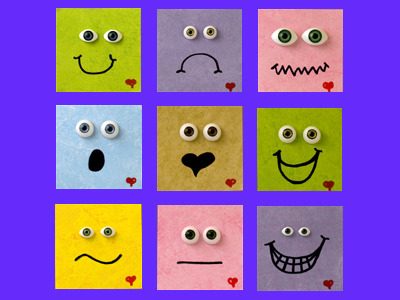Squares are one of the most common 2-D shapes. Triangles, circles and oblongs are also 2-D shapes.

# Describe 2D Shapes

This Math quiz is called 'Describe 2D Shapes' and it has been written by teachers to help you if you are studying the subject at elementary school. Playing educational quizzes is a fun way to learn if you are in the 1st or 2nd grade - aged 6 to 8.

It costs only \$12.50 per month to play this quiz and over 3,500 others that help you with your school work. You can subscribe on the page at Join Us

When talking about 2-dimensional shapes and other related objects, elementary school children will understand that they can use different words to describe their properties. They will use language such as sides, edges, corners and faces and recognize that some shapes have the same name but may look different.

This quiz will familiarize your child with the language used to describe 2-D shapes so that they can accurately name them and other related objects.

Can you recognize the properties of these 2D shapes?

Click on the pictures for a closer look.

1.
The colored part of the eye is a...
rectangle
square
triangle
circle
The iris and pupil are both circles
2.
What shape is this clock face?
A rectangle
A circle
A square
A triangle
All four sides are the same length
3.
A square is a kind of...
sphere
triangle
circle
rectangle
A rectangle has four sides - a square is a kind of rectangle with four equal sides
4.
This triangle is called an equilateral triangle because all its sides and angles are..
different lengths
the same color
the same
Equilateral means equal or the same
5.
This little girl is holding...
a triangle and a circle
a triangle and a square
a circle and a rectangle
a rectangle and a square
A triangle has three straight sides and a circle has one curved side
6.
What shape are the pairs of triangles making?
Oblongs
Circles
Triangles
Squares
Two right triangles pushed together can make a square
7.
How are rectangles different to squares?
They don't have as many corners
They have 4 sides of different lengths
They have two long and two short sides
They have more sides
Squares have 4 sides of equal length
8.
triangle
square
oval
oblong
Even though it is tilted, the shape is still a square
9.
Which shape has the most corners
They all have the same number of corners
The circle
The triangle
The square
The square has four corners, the triangle has three and the circle has no corners
10.
This pattern is made up from rectangles. Which shape wouldn't fit together as well?
Triangles
Circles
Squares
Cubes
Because circles are curved, they don't fit together at all!
Author:  Angela Smith